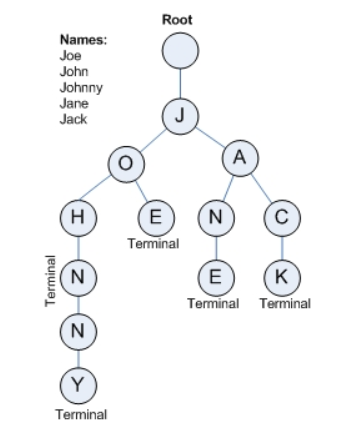# 字典树（前缀树后缀树） - Cache One

### 用途

• 也被称为 前缀树，就是剔除相同的前缀操作，这里看不懂很正常，后面慢慢说
• 搜索提示，讲了下面结构你就理解了

### 结构### 定义数据结构

``````class NodeTree{
// 用数组编号代替字符，会减小搜索查询时间复杂度
NodeTree[] charNode;
// 记录下面子节点被使用个数，为了删除操作
int count = 0;
NodeTree(){
charNode = new NodeTree;
}
}
``````

### 添加（构建）

``````    // 直接添加字符串
public void addString(String s, NodeTree root){
for(int i = 0; i < s.length(); i++){
char c = s.charAt(i);
if(root.charNode[c-'a']==null)
root.charNode[c-'a'] = new NodeTree();
root = root.charNode[c-'a'];
root.count++;
}
}
// 单个添加字符
public NodeTree addChar(char c, NodeTree root){
if(root.charNode[c-'a']==null)
root.charNode[c-'a'] = new NodeTree();
root.count++;
return root.charNode[c-'a'];
}
``````

``````    public boolean findString(String s, NodeTree root){
boolean flag = false;
for(int i = 0; i < s.length(); i++){
char c = s.charAt(i);
if(root.charNode[c-'a']==null)
return flag;
root = root.charNode[c-'a'];
}
return !flag;
}
``````

#### 小测试

``````public class TrimTree {
public static void main(String[] args) {
NodeTree root = new NodeTree();
System.out.println(root.findString("tes", root));
System.out.println(root.findString("test", root));
System.out.println(root.findString("tests", root));
}
}
// 输出
true
true
false
``````